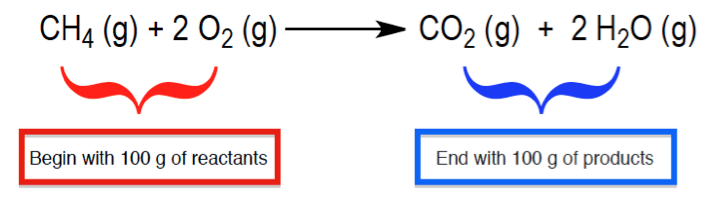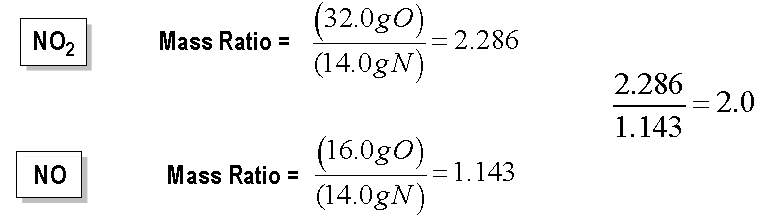Ch.2 - Atoms & ElementsWorksheetSee all chapters
 Ch.1 - Intro to General Chemistry 2hrs & 53mins 0% complete Worksheet Ch.2 - Atoms & Elements 2hrs & 49mins 0% complete Worksheet Ch.3 - Chemical Reactions 3hrs & 25mins 0% complete Worksheet BONUS: Lab Techniques and Procedures 1hr & 38mins 0% complete Worksheet BONUS: Mathematical Operations and Functions 47mins 0% complete Worksheet Ch.4 - Chemical Quantities & Aqueous Reactions 3hrs & 30mins 0% complete Worksheet Ch.5 - Gases 3hrs & 47mins 0% complete Worksheet Ch.6 - Thermochemistry 2hrs & 28mins 0% complete Worksheet Ch.7 - Quantum Mechanics 2hrs & 35mins 0% complete Worksheet Ch.8 - Periodic Properties of the Elements 1hr & 57mins 0% complete Worksheet Ch.9 - Bonding & Molecular Structure 2hrs & 5mins 0% complete Worksheet Ch.10 - Molecular Shapes & Valence Bond Theory 1hr & 31mins 0% complete Worksheet Ch.11 - Liquids, Solids & Intermolecular Forces 3hrs & 40mins 0% complete Worksheet Ch.12 - Solutions 2hrs & 17mins 0% complete Worksheet Ch.13 - Chemical Kinetics 2hrs & 22mins 0% complete Worksheet Ch.14 - Chemical Equilibrium 2hrs & 26mins 0% complete Worksheet Ch.15 - Acid and Base Equilibrium 4hrs & 42mins 0% complete Worksheet Ch.16 - Aqueous Equilibrium 3hrs & 48mins 0% complete Worksheet Ch. 17 - Chemical Thermodynamics 1hr & 44mins 0% complete Worksheet Ch.18 - Electrochemistry 2hrs & 58mins 0% complete Worksheet Ch.19 - Nuclear Chemistry 1hr & 33mins 0% complete Worksheet Ch.20 - Organic Chemistry 3hrs 0% complete Worksheet Ch.22 - Chemistry of the Nonmetals 2hrs & 1min 0% complete Worksheet Ch.23 - Transition Metals and Coordination Compounds 1hr & 54mins 0% complete Worksheet

# Atomic Theory

See all sections
Sections
Periodic Table
Mole Concept
Isotopes
Mass Spectrometry
Subatomic Particles
Atomic Theory
Thomson's Cathode Ray Tube Experiment
Oil Drop Experiment
Gold Foil Experiment
End of Chapter 2 Problems

Modern Atomic Theory states that matter is composed of small, indivisible particles called atoms.

###### Three Laws of Modern Atomic Theory

Concept #1: The Conservation of Matter

Transcript

We already know that the basic functional unit in chemistry is the atom. But we should realize that associated with the atom are theories that scientists from the past developed to better understand how do these atoms interact with one another, as well as their surroundings.
Now, we're going to say according to the Law of Conservation of Mass, in a chemical reaction, any type of chemical reaction, matter can neither be created nor destroyed. The only thing that happens is that matter changes forms.
Here, when I give us an example, we have CH4 gas, which is called methane gas, reacts with oxygen gas to give us CO2 gas plus water. What we mean by this law of conservation of mass, what it means is let's say we started out with 100 grams of these two reactants. On the left side of the arrow, we call these reactants. Let's say we started out with 100 g of it. At the end of our reaction, we formed products. And at the end of the reaction, we should still end up with 100 g.
We also can say that connected to this Law of Conservation of Mass is that we should realize that at the end of our reaction, we should have equal numbers of each individual element. We're going to say on the left side, we have one carbon, we have four hydrogens and we have two, times another two, we have four oxygens.
At the end of our reaction, we should still have the same number of carbons, hydrogens, and oxygens. This also goes in line with the conservation of mass. We have one carbon. We have two times two, we have four hydrogens. Then this two gets distributed, so we have two times one, we have two oxygens here, plus another two oxygens here, so we have four oxygens total.
Whether you're looking at it in terms of grams produced from grams starting with or you're looking at it in terms of, I started out with this number of elements, I should end with the same number of elements—both are staying the same thing.
What you start in a certain quantity, you should end with at the end of the reaction. Matter doesn't get destroyed. If we start out with 100 grams, we shouldn’t end with less than 100 grams. We should only end with 100 grams because we're conserving the mass that we had.

The Law of Conservation of Mass states that matter can neither be created nor destroyed, all that happens is that it changes forms.Concept #2: Law of Definite Proportions

Transcript

A second important atomic theory is we're going to say, according to the law of definite proportions, all samples of a compound, no matter their origin or preparation has the same ratio in terms of their elements. Now, what exactly does this mean? So, here I gave us an example of CO2, we say that this is called carbon dioxide, don't worry about how we came up with that name, we'll learn about naming later on. But carbon dioxide can be formed in various ways, carbon dioxide can be produced from us just exhaling, so let's say I'm exhaling ou CO2 and then let's say we have a car pushing at exhaust, we can also make some CO2 from that as well. Now, that's 2 different sources CO2 is coming from, one is coming from me exhaling out, one is coming from machinery, they're different sources for CO2 but it doesn't matter, at the end of the day we have CO2 produced from both sources and we're going to say, because of that both will have the same mass ratio, that's what the law of definite proportion says, it doesn't matter where the CO2 comes from, all that matter is both of them are CO2 and since they're both CO2 they both have the same mass ratio, and what exactly is this mass ratio? So, let's take a look here, if we look at our periodic table, just look at any periodic table, you'll see that mass of carbon atom there, it's atomic mass is about 12 grams, if you take a look at oxygen, you'll see that oxygen at you periodic table is 16 grams, but we don't have 1 oxygen in our CO2 compound, we have 2 oxygens, so the total weight of oxygen is really 2 times 16 grams, that's why we came up with this 32 grams of oxygen that we put here. Now, the mass ratio is 12 divided by 32, which gives us 3.75. Now, if we said that this CO2 came from me exhaling, doesn't matter, if we have CO2 from the exhaust of a car or from some type of heavy machinery it would still have the same mass ratio, it'd still be 12 grams of carbon over 32 grams of oxygen and it would still have the same mass ratio, that's what the of definite proportion is saying.

No matter where you obtain a compound, whether it’s from a lab experiment or from collection out in the field, the ratio of elements in it will remain constant.

Concept #3: Law of Multiple Proportions

Transcript

The last important atomic theory is we're going to say according to the Law of Multiple Proportions, when two elements, which we're going to say are A and B, form different compounds. Here in this example, our element A will be nitrogen and our element B could be oxygen. And we're going to say the masses of element B that combine with 1 g of A are a ratio of whole numbers. This one is a little bit trickier.
Here we're dealing with two different compounds. We're dealing with NO, which is nitrogen monoxide, and then NO2 which is nitrogen dioxide. We do the mass ratios of both. Nitrogen is 14 g roughly on our periodic table, oxygen is 16. Their mass ratio to each other in NO is 1.143. Now, if we do NO2, since we have two oxygens, it's 16 times 2, which gives us 32. So 32 divided by 14, give us this new number. That's the mass ratio for each of them.
Now, the Law of Multiple Proportions tells me, if I take those mass ratios and I divide them by each other, it should give me back a whole number as an answer. I'm going to take this 2.286 and I divide it by the 1.143 and it spits back to me, 2. So what that's telling me is that we'd have two oxygens, two grams of oxygen for every 1 g of nitrogen. That's really what it's telling me.
The Law of Multiple Proportions for this type of questions, it's going to be a quick type of question that your professor asks you, nothing in too depth where you have to write long paragraphs or anything. As long as you can grasp the basic concepts of each of these, you'll better be able to answer the questions that they're going to ask you.
Then, again, they're not going to be too in depth with those types of questions. So, all that matters is that you understand the most basic parts of these three modern atomic theories. That's the most important thing.

When element A and element B combine they can form different compounds in different ratios to one another. Dividing these different ratios should generate whole number answers.Example #1: A 15.39 g sample of iodine reacts with 62.92 g of chlorine to form iodine pentachloride, ICl5. If iodine pentachloride is the only product formed calculate its mass.

Example #2: Two samples sodium fluoride decompose into their constituent elements. The first sample produces 15.8 kg of sodium and 20.1 kg of fluorine. If the second sample produces 192.0 g of sodium, how many grams of fluorine were also produced?

Practice: Which of the following is an example of the law of multiple proportions?